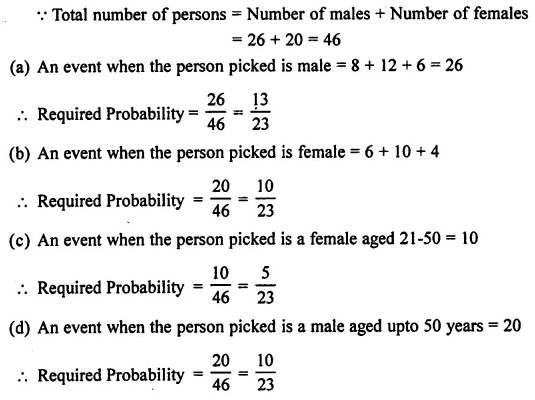# Selina Concise Mathematics Class 7 ICSE Solutions Chapter 22 Probability

## Selina Concise Mathematics Class 7 ICSE Solutions Chapter 22 Probability

Selina Publishers Concise Mathematics Class 7 ICSE Solutions Chapter 22 Probability

### Probability Exercise 22A – Selina Concise Mathematics Class 7 ICSE Solutions

Question 1.
A coin is tossed once. Find the probability of
Solution:Question 2.
A coin is tossed 80 times and the head is obtained 38 times. Now, if a coin tossed once, what will the probability of getting:
(i) a tail
Solution: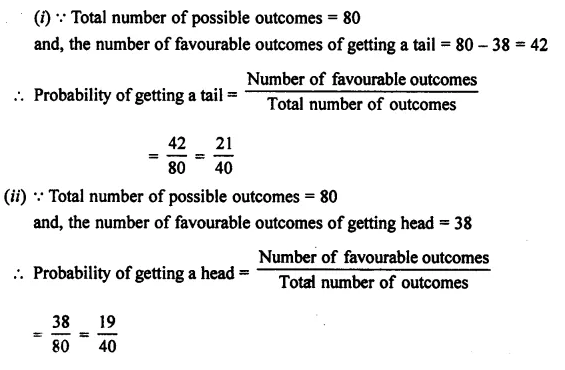Question 3.
A dice is thrown 20 times and the outcomes are noted as shown below :Now a dice is thrown at random, find the probability of getting :
Solution: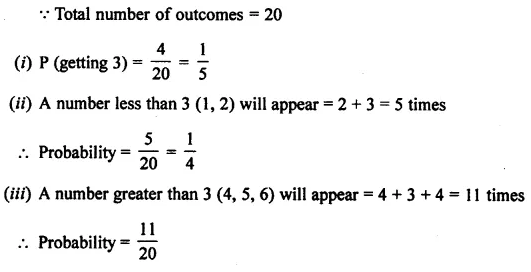Question 4.
A survey of 50 boys showed that 21 like tea while 29 dislike it. Out of these boys, one boy is chosen at random. What is the probability that the chosen boy
(i) likes tea
(ii) dislikes tea
Solution:Question 5.
In a cricket match, a batsman hits a boundary 12 times out of 80 balls he plays, further, if he plays one ball more, what will be the probability that:
(i) he hits a boundary
(ii) he does not hit a boundary
Solution: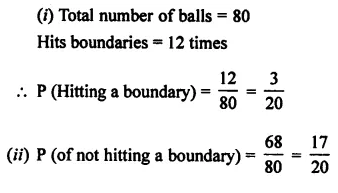Question 6.
There are 8 marbles in a bag with numbers from 1 to 8 marked on each of them. What is the probability of drawing a marble with number
(i) 3
(ii) 7
Solution:Question 7.
Two coins are tossed simultaneously 100 times and the outcomes are as given below: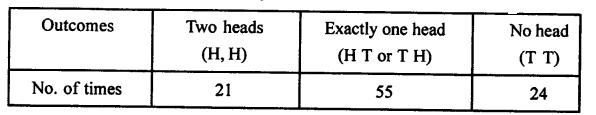If the same pair of coins is tossed again at random, find the probability of getting :
Solution:Question 8.
A bag contains 4 white and 6 black balls,- all of the same shape and same size. A ball is drawn from the bag without looking into the bag. Find the probability that the ball drawn is :
(i) a black ball
(ii) a white ball
(iii) not a black ball
Solution:Question 9.
In a single throw of a dice, find the probability of getting a number:
(i) 4
(ii) 6
(iii) greater than 4
Solution:Question 10.
Hundred identical cards are numbered from 1 to 100. The cards are well shuffled and then a card is drawn. Find the probability that the number on the card drawn is :
(i) 50
(ii) 80
(iii) 40
Solution:### Probability Exercise 22B – Selina Concise Mathematics Class 7 ICSE Solutions

Question 1.
Suppose S is the event that will happen tommorow and P(S) = 0.03.
(i) State in words, the complementary event S’.
(ii) Find P(S’)
Solution: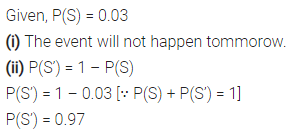Question 2.
Five Students A, B, C, D and E are competing in a long distance race. Each student’s probability of winning the race is given below:
A → 20 %, B → 22 %, C → 7 %, D → 15% and E → 36 %
(i) Who is most likely to win the race ?
(ii) Who is least likely to win the race ?
(iii) Find the sum of probabilities given.
(iv) Find the probability that either A or D will win the race.
(v) Let S be the event that B will win the race.
(a) Find P(S)
(b) State, in words, the complementary event S’.
(c) Find P(S’)
Solution:Question 3.
A Ticket is randomly selected from a basket containing3 green, 4 yellow and 5 blue tickets. Determine the probability of getting:
(i) a green ticket
(ii) a green or yellow ticket.
(iii) an orange ticket.
Solution: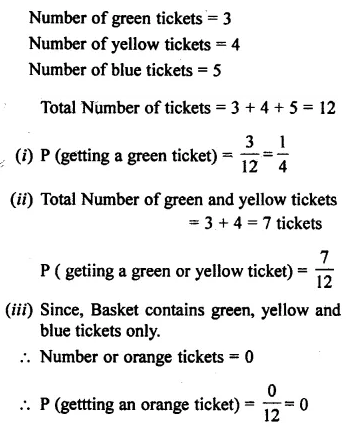Question 4.
Ten cards with numbers 1 to 10 written on them are placed in a bag. A card is chosen from the bag at random. Determine the probability of choosing:
(i) 7
(ii) 9 or 10
(iii) a number greater than 4
(iv) a number less than 6
Solution:Question 5.
A carton contains eight brown and four white eggs. Find the probability that an egg selected at random is :
(i) brown
(ii) white
Solution: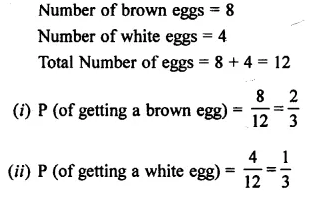Question 6.
A box contains 3 yellow, 4 green and 8 blue tickets. A ticket is chosen at random. Find the probability that the ticket is :
(i) yellow
(ii) green
(iii) blue
(iv) red
(v) not yellow
Solution: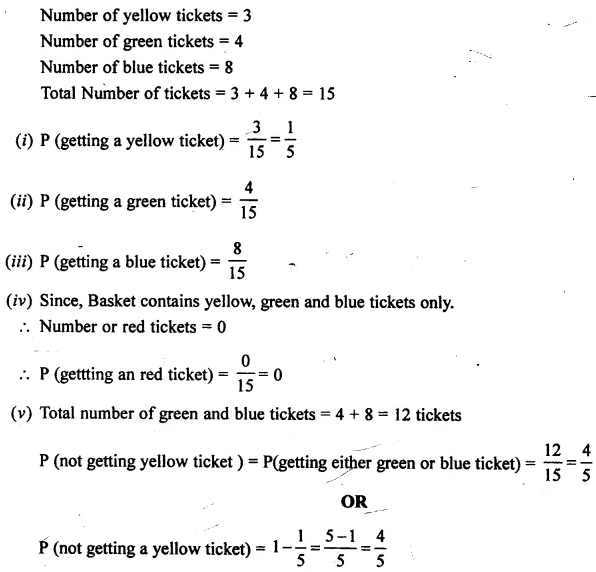Question 7.
The following table shows number of males and number of females of a small locality in different age groups.
If one of the persons, from this locality, is picked at random, what is the probability that
(a) the person picked is a male ?
(b) the person picked is a female ?
(c) the person picked is a female aged 21-50 ?
(d) the person is a male with age upto 50 years?
Solution: